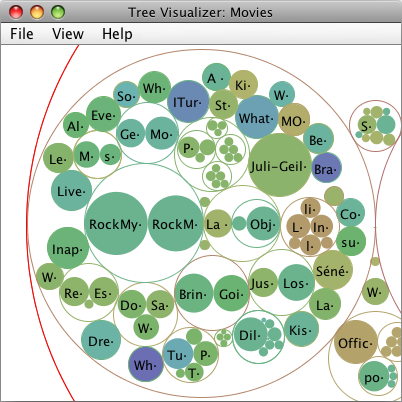This week, I made an other attempt at my project for the visualization of large tree structures. I have implemented a circular treemap. Check it out here.

Implementing a good circle packing algorithm for the circular treemap is very hard. First I tried a simple generative approach, which lais out the circles in a phyllotactic spiral. Phyllotactic spirals are a common pattern found in nature, for example in a marguerite:An algorithm for generating this pattern is only a few lines long:

```for (i = 1; i <= n; i++) {

theta = i * 137.5°;

}```

In this algorithm n is the number of circles, and c is a constant for the spacing between circle centers, radius and theta are the locations of the circles in polar coordinates.

Unfortunately, the phyllotactic spiral only works well, if there are many circles, and all circles have roughly the same size.

I am now using an algorithm, which works with pairs of circles. For this algorithm, we first sort the circles by size. With the three largest circles, we create a tightly packed triangle, by placing the circles in the corners of the triangle, and letting the length of an edge be the sum of the radii of the two circles it connects.

Then we create a list of each possible pairings of these triangles. With a triangle with corners A, B, C, we get six possible pairings: A-B, B-C, C-A, B-A, C-B, A-C. With each subsequent circle, we try to create a new triangle with these pairings. We choose the pairing, which places the subsequent circle closest to the center of the original triangle, and which does not result in an overlap with the cirlces we have already laid out. Once we found the best triangle, for example A-B with D, we add the four additionally possible pairings to the list: A-D, D-B, D-A, B-D. And then, we process the next circle.

This is not an optimal algorithm, but it gives fairly good results. Here is screenshot of a circular treemap generated with it: